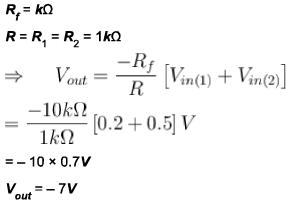Courses

# OP–amp Nat Level – 2

## 10 Questions MCQ Test Topic wise Tests for IIT JAM Physics | OP–amp Nat Level – 2

Description
This mock test of OP–amp Nat Level – 2 for IIT JAM helps you for every IIT JAM entrance exam. This contains 10 Multiple Choice Questions for IIT JAM OP–amp Nat Level – 2 (mcq) to study with solutions a complete question bank. The solved questions answers in this OP–amp Nat Level – 2 quiz give you a good mix of easy questions and tough questions. IIT JAM students definitely take this OP–amp Nat Level – 2 exercise for a better result in the exam. You can find other OP–amp Nat Level – 2 extra questions, long questions & short questions for IIT JAM on EduRev as well by searching above.
*Answer can only contain numeric values
QUESTION: 1

### The output Vo of the ideal Op-Amp circuit shown is the figure is (in volts).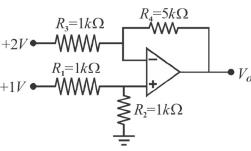Solution: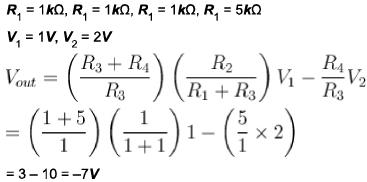*Answer can only contain numeric values
QUESTION: 2

### The circuit shown below represent a non-inverting integrator. For high frequency input (ω →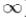) impedance in ohms is?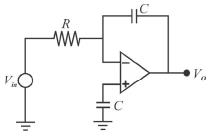Solution:

Input impedance is given as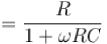For (ω →) input impedance → 0

*Answer can only contain numeric values
QUESTION: 3

### The difference between the bandwidth of the amplifiers given below when both the Not answered Op-Amps have an open loop gain of 100dB and a unity-gain bandwidth of 3MHz (in KHz) is :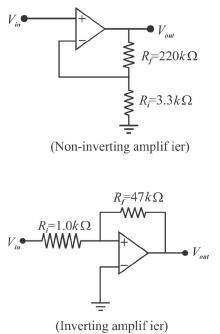Solution:

For non-inverting amplifier, closed loop gain is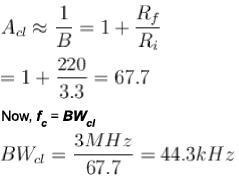For the inverting amplifier, the closed loop gain is.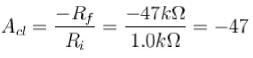Using the absolute value of Acl the closed-loop bandwidth is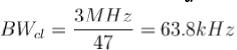Therefore difference in their bandwidth is
= 63.8 - 44.3
= 19.5 kHz

*Answer can only contain numeric values
QUESTION: 4

In the given circuit using ideal Op-Amps, the output voltage (in mV) is :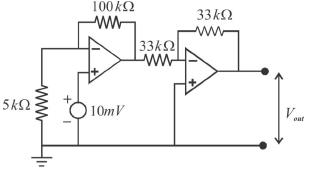Solution: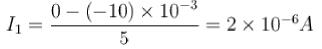Hence, V1= 2x106 x100x103 = 200x10-3V
Since, gain of second stage is -1
⇒ Vout = - 200mV

*Answer can only contain numeric values
QUESTION: 5

The output voltage for the scaling adder in figure shown below is (in volts)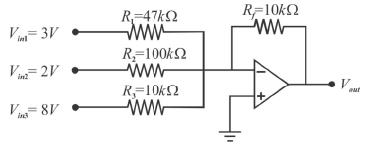Solution: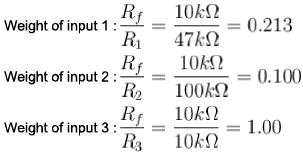The output voltage is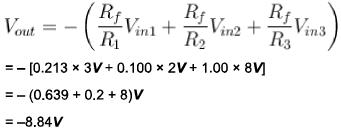*Answer can only contain numeric values
QUESTION: 6

Figure shows a practical integrator with Rs = 30MΩ, RF = 20MΩ and CF = 0.1 μF. If a step (dc) voltage of +3V is applied as input for 0 < t < 4 (t is in seconds), the output voltage is a ramp function. What is the voltage in volts?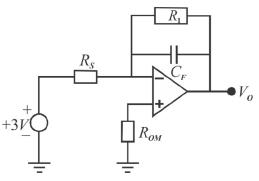Solution: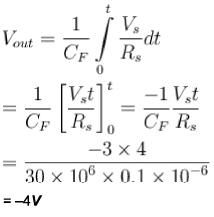*Answer can only contain numeric values
QUESTION: 7

The certain inverting amplifier has a closed loop gain of 25. The Op-Amp has an open-loop gain of 100000. If another Op-Amp with an open loop gain of 200000 is substituted in the configuration, the closed loop gain is?

Solution:

The closed loop gain depends on the configuration and open loop gain is completely independent of it. Hence even if open loop gain is changed, the closed loop gain remains at 25 with the same configuration.

*Answer can only contain numeric values
QUESTION: 8

If the voltage gain for each input of a summing amplifier with a 4.7 kΩ feedback resistor is unity, the input resistors must have a value of (in kΩ?

Solution:

Voltage gain for each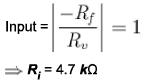*Answer can only contain numeric values
QUESTION: 9

An averaging amplifier has five inputs. Ratio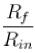must be?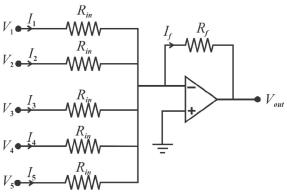Solution: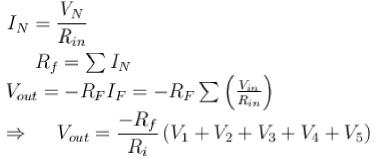Now to average 5 inputs, Rf should be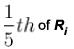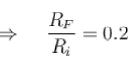*Answer can only contain numeric values
QUESTION: 10

The output voltage for the summing amplifier shown in figure is : (in volts)',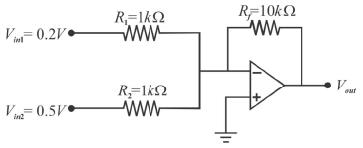Solution: